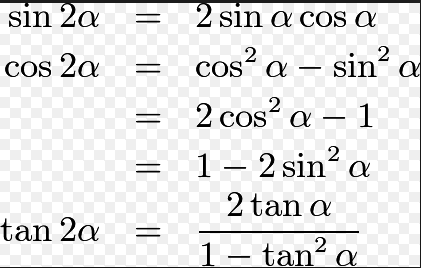#### Letand let, whereThenOption 1)Option 2)Option 3)Option 4)As we leant in

Double Angle Formula -- wherein

These are formulae for double angles.Option 1)This option is incorrect.

Option 2)This option is correct.

Option 3)This option is incorrect.

Option 4)This option is incorrect

#### Vakul